Next: Simulations Up: Ensemble Learning for Independent Previous: Model for the measurements

# Diagonal Gaussian ensemble

Given the measurements, the unknown variables of the model are the source signals, the mixing matrix, the parameters of the noise and source distributions and the hyperparameters. The posterior P is thus a pdf of all these unknown variables. For notational simplicity, we shall sometimes denote these n variables by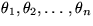.

In order to make the approximation of the posterior pdf computationally tractable, we shall choose the ensemble Q to be a Gaussian pdf with diagonal covariance. The ensemble has twice as many parameters as there are unknown variables in the model because each dimension of the posterior pdf is parametrised by a mean and variance in the ensemble. A hat over a symbol denotes the mean and a tilde the variance of the corresponding variable.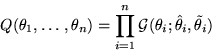The factorised ensemble makes the computation of the Kullback-Leibler information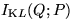simple since the logarithm can be split into a sum of terms: the terms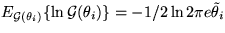(entropies of Gaussian distributions) and terms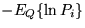, where Pi are the factors of the posterior pdf. Notice that the posterior pdf factorises into simple terms due to the hierarchical structure of the model; the posterior pdf equals to the joint pdf divided by a normalising term.

To see how to compute the terms, let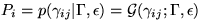.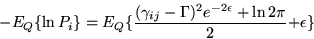(1)
It is easy to show that this equals to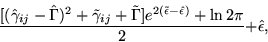since according to the choice of Q, the parameters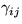,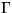and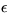have independent Gaussian distributions.

The most difficult terms are the expectations of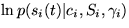. Approximation for the expectation of this form is given in appendix A.

The normalising term in the posterior pdf only depends on those variables which are given, in this case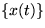, and can therefore be neglected when minimising the Kullback-Leibler information.Next: Simulations Up: Ensemble Learning for Independent Previous: Model for the measurements
Harri Lappalainen
7/10/1998